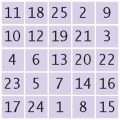Implement the following algorithm to construct magic n-by-n2 squares; it works only if n is odd. Place a 1 in the middle of the bottom row. After k has been placed in the (i, j) square, place k + 1 into the square to the right and down, wrapping around the borders. However, if the square to the right and down has already been filled, or if you are in the lower-right corner, then you must move to the square straight up instead. Here is the 5 × 5 square that you get if you follow this method:Write a program whose input is the number n and whose output is the magic square of order n if n is odd. Implement a class MagicSquare with a constructor that constructs the square and a toString method that returns a representation of the square.

Complete the following file:

### MagicSquare.java

Use the following file:

MagicSquareMaker.java

```import java.util.Scanner;

/**
This class makes a magic square.
*/
public class MagicSquareMaker
{
public static void main(String[] args)
{
Scanner in = new Scanner(System.in);
System.out.print("Enter the size of the square (must be odd): ");
int size = in.nextInt();

if (size % 2 == 1)
{
MagicSquare mySquare = new MagicSquare(size);
System.out.println(mySquare.toString());
}
else
System.out.println("Size must be odd." );
}
}
```